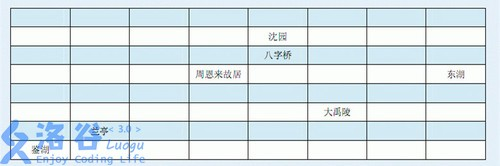# Ⅰ、抛出问题

## 题目描述### Sample Input

4 4
0 1 1 0
2 5 5 1
1 5 5 1
0 1 1 0

### Sample Output

xoox
___o
___o
xoox

# Ⅱ、分析问题

$dp[i][sta]=min_{s\in sta} \{dp[i][s]+dp[i][sta\otimes s]-val[i]\}$

$dp[i][sta]=\min\{dp[j][sta]+val[i](i\to j)\}$

#include<bits/stdc++.h>
#define F(i,j,n) for(register int i=j;i<=n;i++)
#define INF 0x3f3f3f3f
#define ll long long
#define mem(i,j) memset(i,j,sizeof(i))
#define pii pair<int,int>
using namespace std;
struct hahaha{
int x,y,sta;
}pre;
const int dx={0,1,0,-1},dy={1,0,-1,0};//位移数组
int n,m,mp,cnt=0,f,lx,ly;//本题中点的标号按坐标存，即(i,j)表示第一维
queue<pii>q;//SPFA队列
bool vis;//(i,j)这个点是否走过
int datta=0;char chchc=getchar();bool okoko=0;
while(chchc<'0'||chchc>'9'){if(chchc=='-')okoko=1;chchc=getchar();}
while(chchc>='0'&&chchc<='9'){datta=datta*10+chchc-'0';chchc=getchar();}
return okoko?-datta:datta;
}
inline void SPFA(int now_sta){
while(!q.empty()){
int x=q.front().first,y=q.front().second;
vis[x][y]=0;
F(p,0,3){
int tx=x+dx[p],ty=y+dy[p];
if(tx>=1&&tx<=n&&ty>=1&&ty<=m){
if(f[tx][ty][now_sta]>f[x][y][now_sta]+mp[tx][ty]){
f[tx][ty][now_sta]=f[x][y][now_sta]+mp[tx][ty];//转移
pre[tx][ty][now_sta].x=x;
pre[tx][ty][now_sta].y=y;
pre[tx][ty][now_sta].sta=now_sta;//记录一下有哪个状态转移而来，方便处理第二问
if(!vis[tx][ty])
vis[tx][ty]=1,q.push(make_pair(tx,ty));
}
}
}
q.pop();
}
}
inline void dfs(int x,int y,int sta){
if(!pre[x][y][sta].x&&!pre[x][y][sta].y)//如果没有，说明走到了一条分支的尽头，return
return ;
vis[x][y]=1;//标记走过
if(pre[x][y][sta].x==x&&pre[x][y][sta].y==y)//如果是由(x,y)位置的另一个状态转移而来(即主函数里的转移)
dfs(x,y,sta^pre[x][y][sta].sta);//递归寻找补集
dfs(pre[x][y][sta].x,pre[x][y][sta].y,pre[x][y][sta].sta);//寻找周围节点(即SPFA中的转移)
}
int main(){
mem(f,0x3f);
F(i,1,n)
F(j,1,m){
if(!mp[i][j])
f[i][j][1<<(cnt++)]=0,lx=i,ly=j;//随便记录一个标记点记做(lx,ly)
}
F(sta,0,(1<<cnt)-1){//枚举联通状态
F(i,1,n){
F(j,1,m){//枚举坐标
for(int s=sta;s;s=(s-1)&sta){//枚举子集
if(f[i][j][sta]>f[i][j][s]+f[i][j][s^sta]-mp[i][j]){
f[i][j][sta]=f[i][j][s]+f[i][j][s^sta]-mp[i][j];//转移
pre[i][j][sta].x=i;
pre[i][j][sta].y=j;
pre[i][j][sta].sta=s;//记录由哪个状态转移而来
}
}
if(f[i][j][sta]<0x3f3f3f3f)
q.push(make_pair(i,j)),vis[i][j]=1;//如果有值，加入SPFA更新队列
}
}
SPFA(sta);//SPFA暴力更新周围节点
}
mem(vis,0);
printf("%d\n",f[lx][ly][(1<<cnt)-1]);//第一问
dfs(lx,ly,(1<<cnt)-1);//寻找走了哪些点
F(i,1,n)
F(j,1,m){
if(!mp[i][j])//如果是障碍物
printf("x");
else
printf("%c",vis[i][j]==1?'o':'_');//走过就o,没走过就_
printf("\n");
}
return 0;
}
posted @ 2019-03-09 16:43  hzf29721  阅读(...)  评论(...编辑  收藏